# Iterated Function Systems

One of the more common, and more general, ways to generate fractals is through Iterated Function Systems (IFSs). The following text gives an overview of the theory of IFSs, including definitions, key theorems, and some examples. We leave out most proofs, and ignore a number of details. For more complete expositions see one of the references in the Further Reading section.

## Contraction Mappings

Def: A contraction mapping on the metric space (X,d) is a mapping f:X->X from the metric space into itself, such that: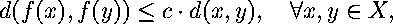for some real constant 0<= c <1, the contractivity factor.

Theorem: (The Contraction Mapping Theorem) Let f:X->X be a contraction mapping on a complete metric space (X,d). Then f has exactly one fixed point, a, in X, and: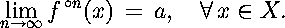Contraction mappings are the elementary building blocks of IFSs, but they are un-interesting by themselves (as seen by the above theorem).

## Iterated Function Systems

Def: An (hyperbolic) iterated function system is a metric space (X,d) together with a finite set of contraction mappings on that space. The notation for such an iterated function system (IFS) is:where each wn is a contraction mapping. We define the contractivity factor of the system to be: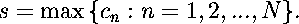Here cn is the contraction factor for wn.

Def: Given a metric space (X,d), we define another metric space (H(X),h(d)). Where H(X) is the set of all nonempty compact subsets of X, and h(d) is the Hausdorf distance between two elements of H(X). In rough terms, the Hausdorff distance between two sets A and B is d if every point of A is within d of some point of B and vice versa.

This metric space (H,h) is in some sense the natural space in which fractals live. In a general way a fractal is an element of this space. However, this is a mathematical abstraction of our intuitive ideas about what a fractal should be (since H includes lot's of normal geometric objects as well.)

Theorem: Let {X; wn, n=1,2,...,N} be a hyperbolic iterated function system on the metric space (X,d) with contractivity factor s. Then the map W:H(X)->H(X) defined by: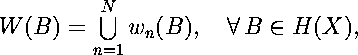whereis a contraction mapping on (H(X),h(d)) with contractivity factor s. Further, the unique fixed point, A, of W is given by: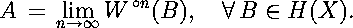Note that a point of H is actually a non-empty compact set of the original space X. This set A is called the attractor of the IFS.

It is the attractors of IFSs, which live in H(X), which are really fractals. Indeed, almost all of the well known fractals, as well as many less well known ones, are the attractors of appropriate IFSs. See some examples generated using Fractalina.

## Finding the Attractor of an IFS

Once an IFS has been defined it is usually necessary to compute the attractor. When the transformations of the IFS can be represented as a matrix transformation on a vector plus a translation, there are two fairly simple ways to compute the attractor: the deterministic algorithm, and the random iteration algorithm.

### The deterministic algorithm:

The deterministic algorithm for finding the attractor of an IFS is the straightfroward application of the map W( ), defined above. That is, an iteration consists of applying each transformation to the current set, and then taking the union of the resulting sets. The limit of this process gives you the attractor of the IFS, as shown above.

Below is an applet that implements the deterministic algorithm for the IFS:Notice that the initial set can vary widely, but the result converges rapidly to the attractor of the IFS. (The attractor of this IFS is the well known Sierpinski triangle.)

To chose the initial set drag in the window, then use the Iterate button to step through iterations on that set.

Oops! Your browser doesn't support Java!

## Continuous Dependence on Parameters

Theorem: Let (X,d) be a metric space, and let {X; wn} be a hyperbolic IFS. Let wn depend continuously on a parameter p (p an element of a compact metric space), for each n. Then the attractor of the IFS, A(p), depends continuously on p.

This theorem provides the mathematical basis for animations of IFSs (in particular for Franimate!). The effect implied here has been called "blowing in the wind", because an imaginary fractal tree can be made to blow in an imaginary mathematical breeze, by continuously varying a parameter to the IFS of the "tree".IFSoft Home PageThe Geometry Center Home Page